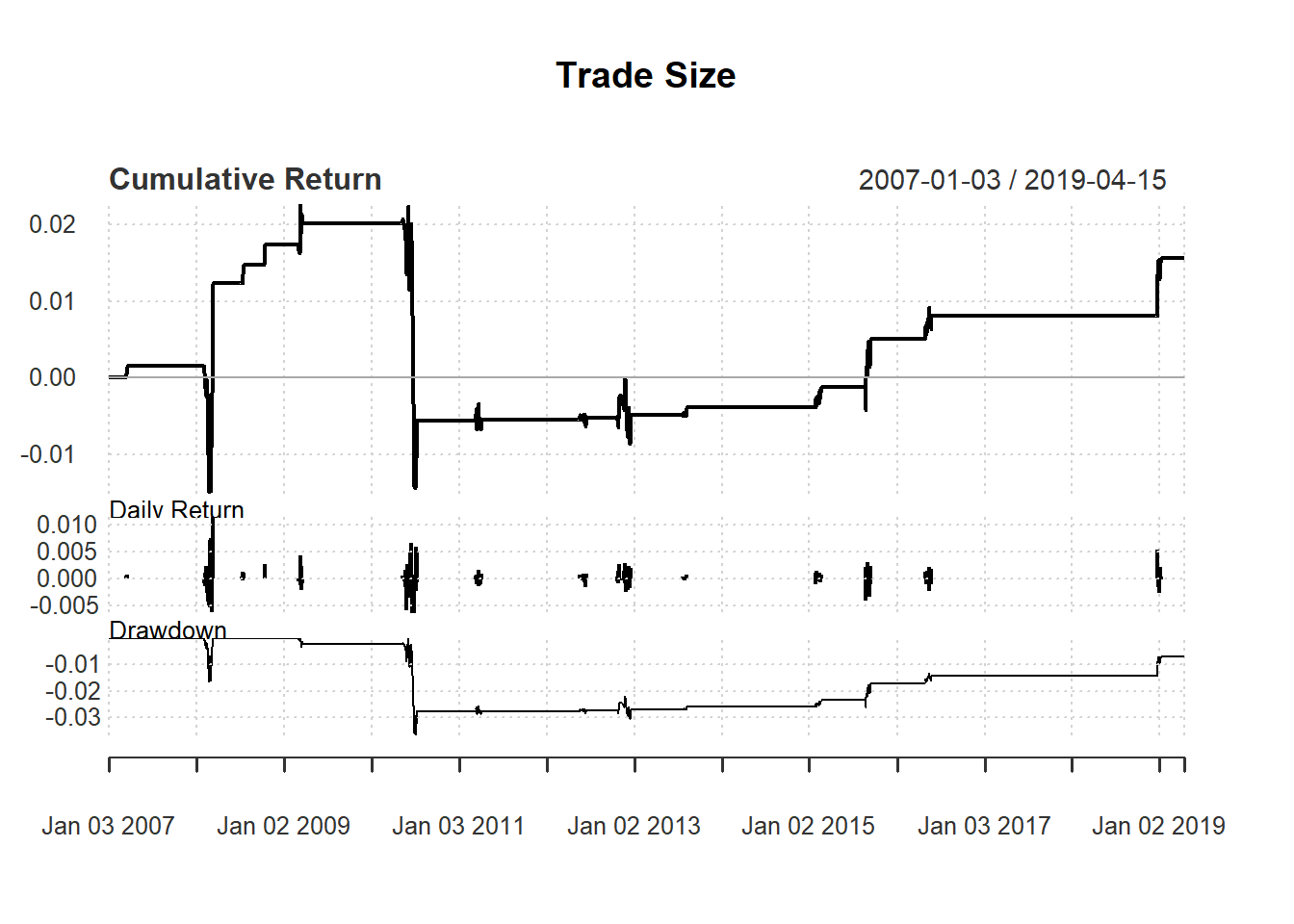Wealth: 1 million

Test the following strategy based on 14-day RSI :

• Buy one more unit if RSI <30.

• Keep buying the same if 30 < RSI < 50

• Stop trading if RSI >= 50

To take trade size into account, we need to keep track of wealth:

qty <-1000
day <-14

signal <- c()    #trade signal with size
signal[1:(day+1)] <- 0

price <- Cl(MSFT)

wealth <-c()
wealth[1:(day+1)] <- 1000000

return<-c()
return[1:(day+1)] <- 0

profit <-c()
profit[1:(day+1)] <- 0

We now generate trading signal with size:

rsi <- RSI(price, day)  #rsi is the lag of RSI
for (i in (day+1): length(price)){
if (rsi[i] < 30){  #buy one more unit if rsi < 30
signal[i] <- signal[i-1]+1
} else if (rsi[i] < 50){  #no change if rsi < 50
signal[i] <- signal[i-1]
} else {         #sell  if rsi > 50
signal[i] <- 0
}
}
signal<-reclass(signal,price)

Close <- Cl(MSFT)
Open <- Op(MSFT)
charts.PerformanceSummary(ret3, main="Trade Size")